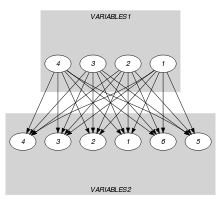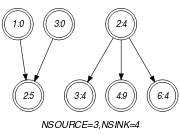## 5.77. common_modulo

Origin

Derived from $\mathrm{𝚌𝚘𝚖𝚖𝚘𝚗}$.

Constraint

$\mathrm{𝚌𝚘𝚖𝚖𝚘𝚗}_\mathrm{𝚖𝚘𝚍𝚞𝚕𝚘}\left(\mathrm{𝙽𝙲𝙾𝙼𝙼𝙾𝙽}\mathtt{1},\mathrm{𝙽𝙲𝙾𝙼𝙼𝙾𝙽}\mathtt{2},\mathrm{𝚅𝙰𝚁𝙸𝙰𝙱𝙻𝙴𝚂}\mathtt{1},\mathrm{𝚅𝙰𝚁𝙸𝙰𝙱𝙻𝙴𝚂}\mathtt{2},𝙼\right)$

Arguments
 $\mathrm{𝙽𝙲𝙾𝙼𝙼𝙾𝙽}\mathtt{1}$ $\mathrm{𝚍𝚟𝚊𝚛}$ $\mathrm{𝙽𝙲𝙾𝙼𝙼𝙾𝙽}\mathtt{2}$ $\mathrm{𝚍𝚟𝚊𝚛}$ $\mathrm{𝚅𝙰𝚁𝙸𝙰𝙱𝙻𝙴𝚂}\mathtt{1}$ $\mathrm{𝚌𝚘𝚕𝚕𝚎𝚌𝚝𝚒𝚘𝚗}\left(\mathrm{𝚟𝚊𝚛}-\mathrm{𝚍𝚟𝚊𝚛}\right)$ $\mathrm{𝚅𝙰𝚁𝙸𝙰𝙱𝙻𝙴𝚂}\mathtt{2}$ $\mathrm{𝚌𝚘𝚕𝚕𝚎𝚌𝚝𝚒𝚘𝚗}\left(\mathrm{𝚟𝚊𝚛}-\mathrm{𝚍𝚟𝚊𝚛}\right)$ $𝙼$ $\mathrm{𝚒𝚗𝚝}$
Restrictions
 $\mathrm{𝙽𝙲𝙾𝙼𝙼𝙾𝙽}\mathtt{1}\ge 0$ $\mathrm{𝙽𝙲𝙾𝙼𝙼𝙾𝙽}\mathtt{1}\le |\mathrm{𝚅𝙰𝚁𝙸𝙰𝙱𝙻𝙴𝚂}\mathtt{1}|$ $\mathrm{𝙽𝙲𝙾𝙼𝙼𝙾𝙽}\mathtt{2}\ge 0$ $\mathrm{𝙽𝙲𝙾𝙼𝙼𝙾𝙽}\mathtt{2}\le |\mathrm{𝚅𝙰𝚁𝙸𝙰𝙱𝙻𝙴𝚂}\mathtt{2}|$ $\mathrm{𝚛𝚎𝚚𝚞𝚒𝚛𝚎𝚍}$$\left(\mathrm{𝚅𝙰𝚁𝙸𝙰𝙱𝙻𝙴𝚂}\mathtt{1},\mathrm{𝚟𝚊𝚛}\right)$ $\mathrm{𝚛𝚎𝚚𝚞𝚒𝚛𝚎𝚍}$$\left(\mathrm{𝚅𝙰𝚁𝙸𝙰𝙱𝙻𝙴𝚂}\mathtt{2},\mathrm{𝚟𝚊𝚛}\right)$ $𝙼>0$
Purpose

$\mathrm{𝙽𝙲𝙾𝙼𝙼𝙾𝙽}\mathtt{1}$ is the number of variables of the collection of variables $\mathrm{𝚅𝙰𝚁𝙸𝙰𝙱𝙻𝙴𝚂}\mathtt{1}$ taking a value situated in an equivalence class (congruence modulo a fixed number $𝙼$) derived from the values assigned to the variables of $\mathrm{𝚅𝙰𝚁𝙸𝙰𝙱𝙻𝙴𝚂}\mathtt{2}$ and from $𝙼$.

$\mathrm{𝙽𝙲𝙾𝙼𝙼𝙾𝙽}\mathtt{2}$ is the number of variables of the collection of variables $\mathrm{𝚅𝙰𝚁𝙸𝙰𝙱𝙻𝙴𝚂}\mathtt{2}$ taking a value situated in an equivalence class (congruence modulo a fixed number $𝙼$) derived from the values assigned to the variables of $\mathrm{𝚅𝙰𝚁𝙸𝙰𝙱𝙻𝙴𝚂}\mathtt{1}$ and from $𝙼$.

Example
$\left(3,4,〈0,4,0,8〉,〈7,5,4,9,2,4〉,5\right)$

In the example, the last argument $𝙼=5$ defines the equivalence classes $a\equiv 0\left(mod5\right)$, $a\equiv 1\left(mod5\right)$, $a\equiv 2\left(mod5\right)$, $a\equiv 3\left(mod5\right)$, and $a\equiv 4\left(mod5\right)$ where $a$ is an integer. As a consequence the items of collection $〈0,4,0,8〉$ respectively correspond to the equivalence classes $a\equiv 0\left(mod5\right)$, $a\equiv 4\left(mod5\right)$, $a\equiv 0\left(mod5\right)$, and $a\equiv 3\left(mod5\right)$. Similarly the items of collection $〈7,5,4,9,2,4〉$ respectively correspond to the equivalence classes $a\equiv 2\left(mod5\right)$, $a\equiv 0\left(mod5\right)$, $a\equiv 4\left(mod5\right)$, $a\equiv 4\left(mod5\right)$, $a\equiv 2\left(mod5\right)$, and $a\equiv 4\left(mod5\right)$. The $\mathrm{𝚌𝚘𝚖𝚖𝚘𝚗}_\mathrm{𝚖𝚘𝚍𝚞𝚕𝚘}$ constraint holds since:

• Its first argument $\mathrm{𝙽𝙲𝙾𝙼𝙼𝙾𝙽}\mathtt{1}=3$ is the number of equivalence classes associated with the items of collection $〈0,4,0,8〉$ that also correspond to equivalence classes associated with $〈7,5,4,9,2,4〉$.

• Its second argument $\mathrm{𝙽𝙲𝙾𝙼𝙼𝙾𝙽}\mathtt{2}=4$ is the number of equivalence classes associated with the items of collection $〈7,5,4,9,2,4〉$ that also correspond to equivalence classes associated with $〈0,4,0,8〉$.

Typical
 $|\mathrm{𝚅𝙰𝚁𝙸𝙰𝙱𝙻𝙴𝚂}\mathtt{1}|>1$ $\mathrm{𝚛𝚊𝚗𝚐𝚎}$$\left(\mathrm{𝚅𝙰𝚁𝙸𝙰𝙱𝙻𝙴𝚂}\mathtt{1}.\mathrm{𝚟𝚊𝚛}\right)>1$ $|\mathrm{𝚅𝙰𝚁𝙸𝙰𝙱𝙻𝙴𝚂}\mathtt{2}|>1$ $\mathrm{𝚛𝚊𝚗𝚐𝚎}$$\left(\mathrm{𝚅𝙰𝚁𝙸𝙰𝙱𝙻𝙴𝚂}\mathtt{2}.\mathrm{𝚟𝚊𝚛}\right)>1$ $𝙼>1$ $𝙼<$$\mathrm{𝚖𝚊𝚡𝚟𝚊𝚕}$$\left(\mathrm{𝚅𝙰𝚁𝙸𝙰𝙱𝙻𝙴𝚂}\mathtt{1}.\mathrm{𝚟𝚊𝚛}\right)$ $𝙼<$$\mathrm{𝚖𝚊𝚡𝚟𝚊𝚕}$$\left(\mathrm{𝚅𝙰𝚁𝙸𝙰𝙱𝙻𝙴𝚂}\mathtt{2}.\mathrm{𝚟𝚊𝚛}\right)$
Symmetries
• Arguments are permutable w.r.t. permutation $\left(\mathrm{𝙽𝙲𝙾𝙼𝙼𝙾𝙽}\mathtt{1},\mathrm{𝙽𝙲𝙾𝙼𝙼𝙾𝙽}\mathtt{2}\right)$ $\left(\mathrm{𝚅𝙰𝚁𝙸𝙰𝙱𝙻𝙴𝚂}\mathtt{1},\mathrm{𝚅𝙰𝚁𝙸𝙰𝙱𝙻𝙴𝚂}\mathtt{2}\right)$ $\left(𝙼\right)$.

• Items of $\mathrm{𝚅𝙰𝚁𝙸𝙰𝙱𝙻𝙴𝚂}\mathtt{1}$ are permutable.

• Items of $\mathrm{𝚅𝙰𝚁𝙸𝙰𝙱𝙻𝙴𝚂}\mathtt{2}$ are permutable.

• An occurrence of a value $u$ of $\mathrm{𝚅𝙰𝚁𝙸𝙰𝙱𝙻𝙴𝚂}\mathtt{1}.\mathrm{𝚟𝚊𝚛}$ can be replaced by any other value $v$ such that $v$ is congruent to $u$ modulo $𝙼$.

• An occurrence of a value $u$ of $\mathrm{𝚅𝙰𝚁𝙸𝙰𝙱𝙻𝙴𝚂}\mathtt{2}.\mathrm{𝚟𝚊𝚛}$ can be replaced by any other value $v$ such that $v$ is congruent to $u$ modulo $𝙼$.

Arg. properties
• Functional dependency: $\mathrm{𝙽𝙲𝙾𝙼𝙼𝙾𝙽}\mathtt{1}$ determined by $\mathrm{𝚅𝙰𝚁𝙸𝙰𝙱𝙻𝙴𝚂}\mathtt{1}$, $\mathrm{𝚅𝙰𝚁𝙸𝙰𝙱𝙻𝙴𝚂}\mathtt{2}$ and $𝙼$.

• Functional dependency: $\mathrm{𝙽𝙲𝙾𝙼𝙼𝙾𝙽}\mathtt{2}$ determined by $\mathrm{𝚅𝙰𝚁𝙸𝙰𝙱𝙻𝙴𝚂}\mathtt{1}$, $\mathrm{𝚅𝙰𝚁𝙸𝙰𝙱𝙻𝙴𝚂}\mathtt{2}$ and $𝙼$.

specialisation: $\mathrm{𝚌𝚘𝚖𝚖𝚘𝚗}$ ($\mathrm{𝚟𝚊𝚛𝚒𝚊𝚋𝚕𝚎}\mathrm{mod}\mathrm{𝚌𝚘𝚗𝚜𝚝𝚊𝚗𝚝}$ replaced by $\mathrm{𝚟𝚊𝚛𝚒𝚊𝚋𝚕𝚎}$).

Keywords
Arc input(s)

$\mathrm{𝚅𝙰𝚁𝙸𝙰𝙱𝙻𝙴𝚂}\mathtt{1}$ $\mathrm{𝚅𝙰𝚁𝙸𝙰𝙱𝙻𝙴𝚂}\mathtt{2}$

Arc generator
$\mathrm{𝑃𝑅𝑂𝐷𝑈𝐶𝑇}$$↦\mathrm{𝚌𝚘𝚕𝚕𝚎𝚌𝚝𝚒𝚘𝚗}\left(\mathrm{𝚟𝚊𝚛𝚒𝚊𝚋𝚕𝚎𝚜}\mathtt{1},\mathrm{𝚟𝚊𝚛𝚒𝚊𝚋𝚕𝚎𝚜}\mathtt{2}\right)$

Arc arity
Arc constraint(s)
$\mathrm{𝚟𝚊𝚛𝚒𝚊𝚋𝚕𝚎𝚜}\mathtt{1}.\mathrm{𝚟𝚊𝚛}\mathrm{mod}𝙼=\mathrm{𝚟𝚊𝚛𝚒𝚊𝚋𝚕𝚎𝚜}\mathtt{2}.\mathrm{𝚟𝚊𝚛}\mathrm{mod}𝙼$
Graph property(ies)
 $•$$\mathrm{𝐍𝐒𝐎𝐔𝐑𝐂𝐄}$$=\mathrm{𝙽𝙲𝙾𝙼𝙼𝙾𝙽}\mathtt{1}$ $•$$\mathrm{𝐍𝐒𝐈𝐍𝐊}$$=\mathrm{𝙽𝙲𝙾𝙼𝙼𝙾𝙽}\mathtt{2}$

Graph class
 $•$$\mathrm{𝙰𝙲𝚈𝙲𝙻𝙸𝙲}$ $•$$\mathrm{𝙱𝙸𝙿𝙰𝚁𝚃𝙸𝚃𝙴}$ $•$$\mathrm{𝙽𝙾}_\mathrm{𝙻𝙾𝙾𝙿}$

Graph model

Parts (A) and (B) of Figure 5.77.1 respectively show the initial and final graph associated with the Example slot. Since we use the $\mathrm{𝐍𝐒𝐎𝐔𝐑𝐂𝐄}$ and $\mathrm{𝐍𝐒𝐈𝐍𝐊}$ graph properties, the source and sink vertices of the final graph are stressed with a double circle. Since the graph has only 3 sources and 4 sinks the variables $\mathrm{𝙽𝙲𝙾𝙼𝙼𝙾𝙽}\mathtt{1}$ and $\mathrm{𝙽𝙲𝙾𝙼𝙼𝙾𝙽}\mathtt{2}$ are respectively equal to 3 and 4. Note that the vertices corresponding to the variables that take values 8, 7 or 2 were removed from the final graph since there is no arc for which the associated arc constraint holds.

##### Figure 5.77.1. Initial and final graph of the $\mathrm{𝚌𝚘𝚖𝚖𝚘𝚗}_\mathrm{𝚖𝚘𝚍𝚞𝚕𝚘}$ constraint(a) (b)